### 1.基本概念以及术语：

• 数据：信息的载体,是 描述客观事物 属性的数、字符及所有能输入到计算机中并被计算机程序识别和处理的 符号的集合
• 数据对象：具有相同性质的数据元素的集合,是数据的个子集
• 数据元素： 数据的基本单位 ,通常作为个整体进行考虑和处理
• 数据项：构成数据元素的不可分割的 最小单位

#### 1.1数据

• 原子类型：值集合+操作（int、char、float等）
• 结构类型：结构的集合+操作（list、map、set等）
• 抽象数据类型ADT：抽象数据类型抽象数据类型(ADT)是指一个数学模型以及定义在该模型上的一组操作。抽象数据类型的定义仅取决于它的一组逻辑特性,而与其在计算机内部如何表示和实现无关。通常用(数据对象、数据关系、基本操作集)这样的三元组来表示抽象数据类型。

#### 1.2结构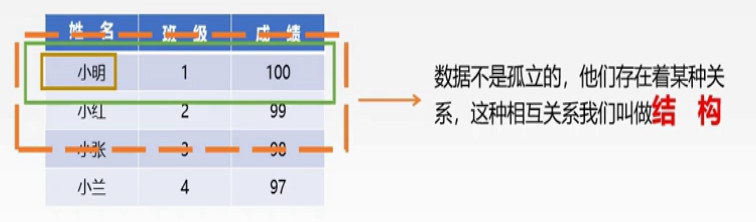• 逻辑结构
• 物理结构（存储结构）
• 数据的运算

#### 1.5逻辑结构

逻辑结构是指数据元素之间的逻辑关系即从逻辑关系上描述数据。它与数据的存储无关,是独立于计算机的。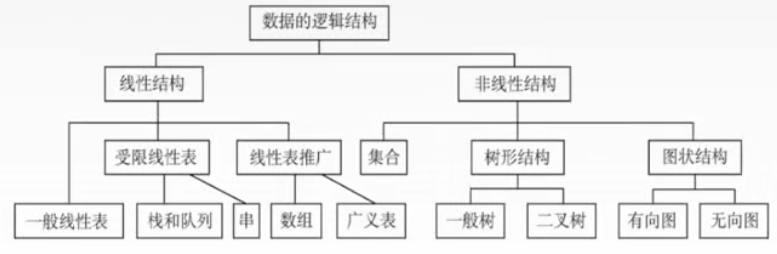• 集合 结构中的数据元素之间除了“同属于一个集合”的关系外,别无其他关系。类似于数学上的集合
• 线性结构 结构中的数据元素之间只存在一对一的关系。比如排队
• 树形结构 结构中的数据元素之间存在一对多的关系。比如家族族谱
• 图状结构或网状结构 结构中的数据元素之间存在多对多的关系。比如地图

#### 1.6物理结构（存储结构）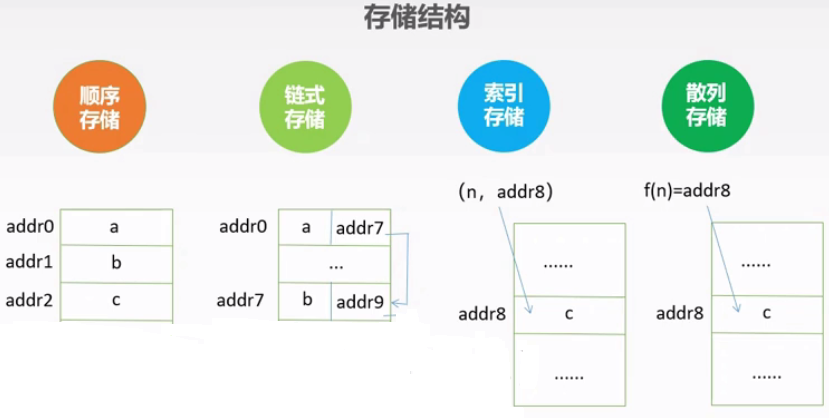• 顺序存储：存储的物理位置相邻。(ps.理位置即信息在计算机中的位置。)
• 链接存储：存储的物理位置未必相邻,通过记录相邻元素的物理位置来找到相邻元素。
• 索引存储：类似于目录，通过索引查询。
• 散列存储：通过关键字直接计算出元素的物理地址(以后详解)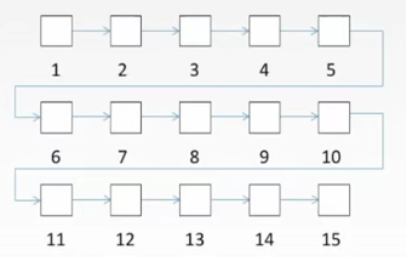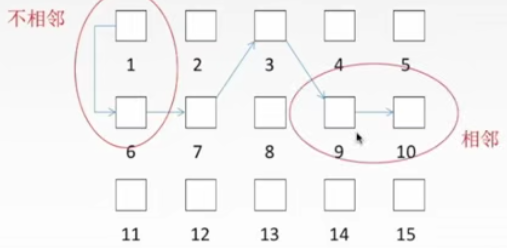#### 1.7数据的运算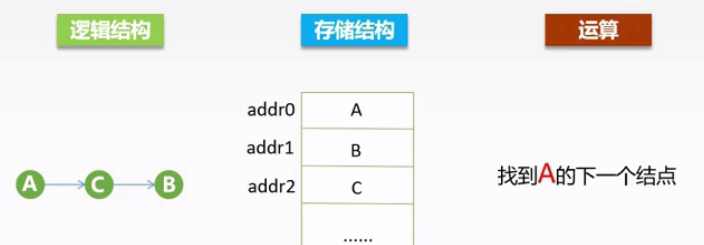### 2.算法&算法评价

#### 2.2算法特性

• 有穷性：一个算法必须在执行有穷步后结束，并且每步操作都在有穷时间内完成。
• 可行性：一个算法必须是可行的，算法中描述的操作是可以实现的。
• 确定性：算法中每一条指令、每一条语句都必须有确定的含义，同样的输入，必须要得到同样的输出。
• 输入：一个算法必须要有零个或者多个输入。
• 输出：一个算法必须要有零个或者多个输出。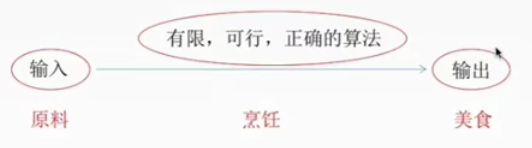#### 2.3算法效率的度量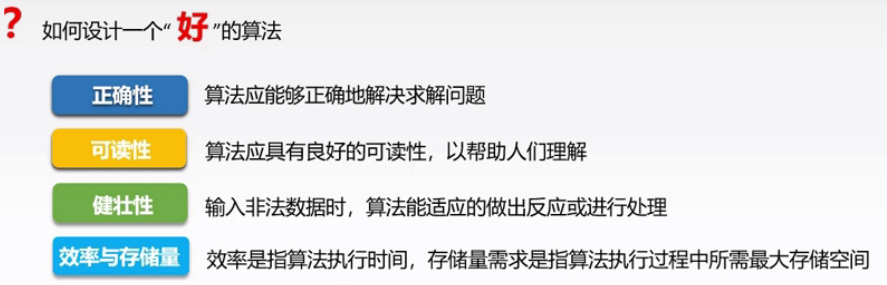时间复杂度:

• 它用来衡量算法随着问题规模增大,算法执行时间增长的快慢。·
• 时间复杂度是问题规模的函数:记作T(n),时间复杂度主要分析T(n)的数量级
• T(n)=O(f(n)),大O记法,f(n)是算法中基本运算的频度,一般我们考虑最坏情况下的时间复杂度。

计算方法:取算法时间增长最快的那个函数项,把它的系数改为1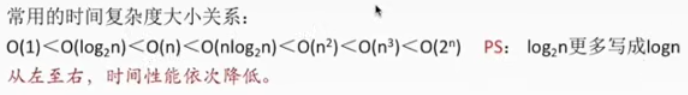O(1)<O(log~2~^n^)<O(n)<O(nlog~2~^n^)<O(n^2^)<O(n^3^)<O(2^n^)<O(n!)<O(n^n^)


空间复杂度:

• 它用来衡量算法随着问题规模增大,算法所需空间的增长的快慢:
• 是问题规模的函数:S(n)=0(g(n))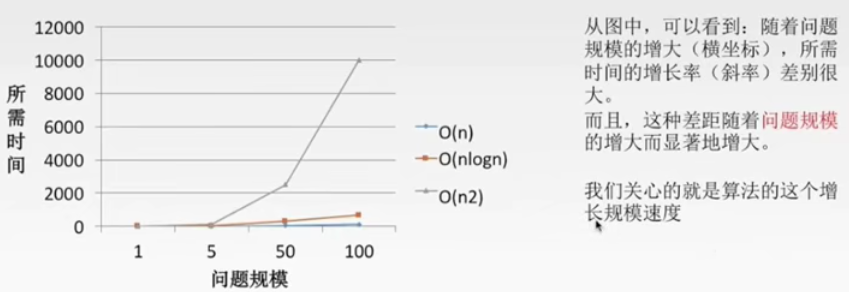#### 2.4时间复杂度

int sum=0;  //执行1次
for(inti=0;i<=n;i++){  //int i=0执行一次，i<n执行n+1次，i++执行n+1次
sum=sum+i;
}


• 复杂度是关于增长率的,所以可以直接忽视常数项，系数化为1
• 一般可以直接关注循环段基本操作语句(示例中的sum=sum+i)的执行次数

直接关注循环体的执行次数,设为k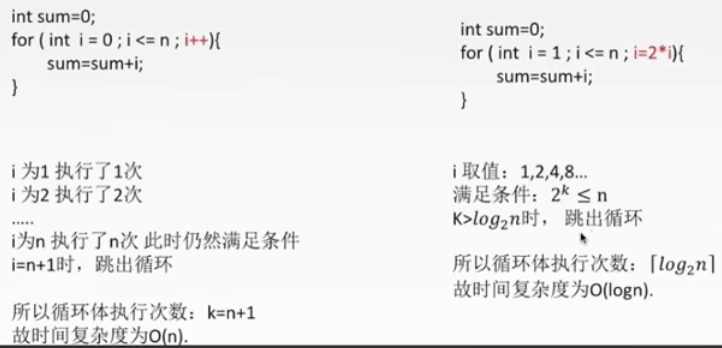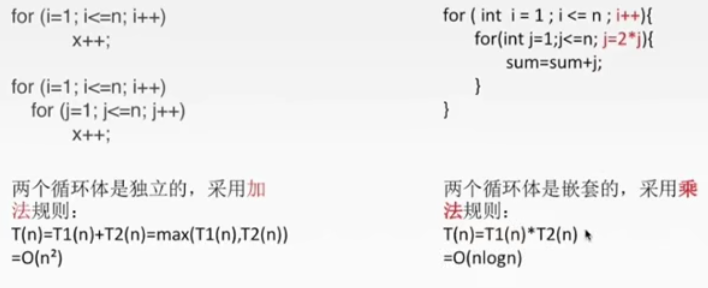#### 2.5空间复杂度

• 辅助空间:除了存储算法本身的指令、常数、变量和输入数据外,还需要存储对数据操作的存储单元。
• 算法原地工作是指算法所需的辅助空间是常量,即O(1)。
• 常见的是O(1),O(n)较多

#### 欢迎关注公众号 理木客 更多精彩等你发现